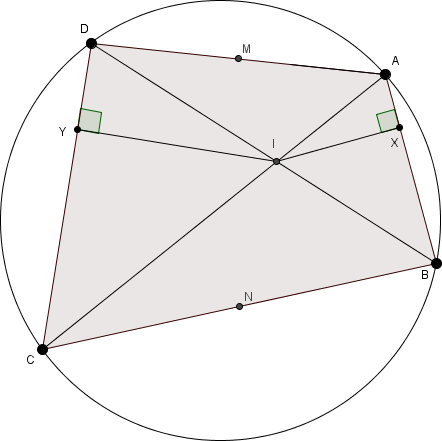# Hidden Similarity

Geometry Level 1The points $A, B, C$ and $D$ are ordered clockwise on a circle.

$M$ and $N$ are the midpoints of $AD$ and $BC$, respectively.

$I$ is the intersection of $AC$ and $BD$.

$X$ and $Y$, are perpendiculars on $AB$ and $CD$ respectively, which pass through $I$.

Is $MXNY$ always a kite?

×